# Kagome - The development of a pattern

Tuesday, 24 April 2018  |  Paul Stephenson

## Introduction

We use the same language to describe uniform tilings and polyhedra: Platonic if all the regular polygons are the same, Archimedean if there's a mixture, and a tiling - like the kagome pattern, 3.6.3.6 - can be thought of as an infinite polyhedron. What controls the size of the polyhedron is the angular defect, d, the difference from 360°, at each vertex. They total 720°, so, if there are v vertices, vd = 720°.

## From 3.6.3.6 to 3.5.3.5

We start with 3.6.3.6 with each '3' in blue and each '6' divided into 6 yellow '3s'. If we remove one yellow triangle from each hexagon and the necessary blue triangles in a patch of the right size, we have the net for a solid whose angular defect is 60°, and number of vertices therefore 720°/60° = 12: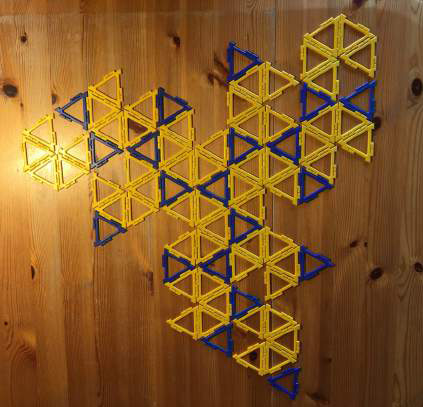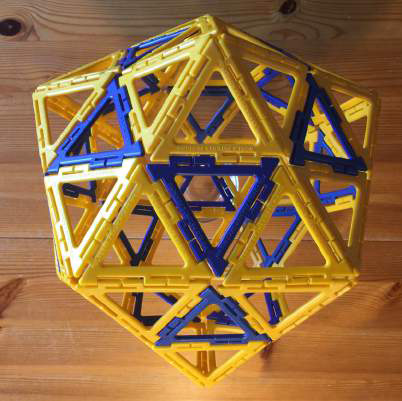This is the icosahedron. It has a feature we shall find throughout: a blue triangle at the centre of each face. What were straight lines in the tiling are polygons in the solid. If we inscribe the solid in a sphere, such polygons are circumscribed by great circles. We shall call these polygons 'equatorial' sections of the solid. We show one such polygon, a 10-gon, in green: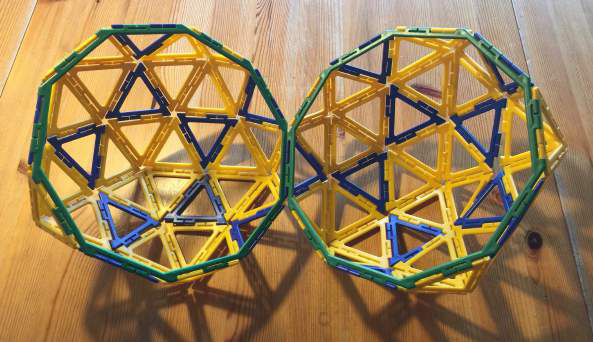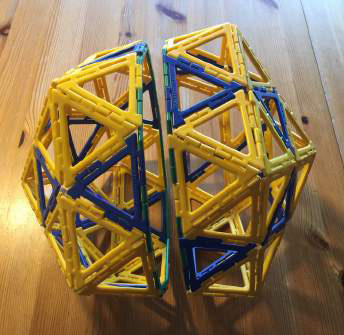There is one for each pair of opposite vertices, so 12/2 = 6 in all.

The yellow triangles form pentagonal pyramids with their apices at the vertices of the icosahedron. If we cut these off, we can replace them with pentagons and we have the solid 3.5.3.5: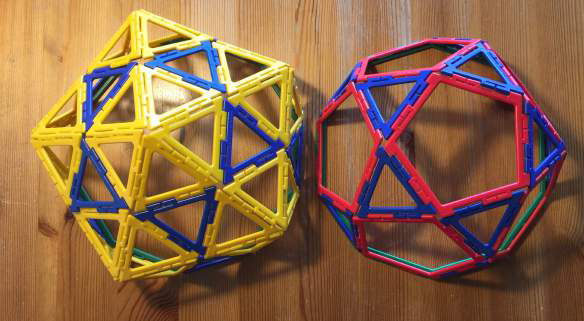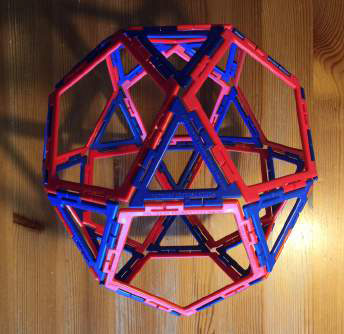There are 20 triangles - one for each face of the icosahedron, and 12 pentagons - one for each vertex of the icosahedron. We can work out the number of vertices in two ways.

There are 5 for each of the 12 pentagons, but two pentagons share a vertex, total 12 x 5/2 = 30.

There are 3 for each of the 20 triangles, but two triangles share a vertex, total 20 x 3/2 = 30.

The angle defect is therefore 720°/30 = 24°.

## From 3.5.3.5 to 3.4.3.4

We go back to our tiling and remove a second yellow triangle from each hexagon, and the necessary blue triangles to form the net of a solid which has an angle defect of 120°, and therefore 720°/120° = 6 vertices. The result is an octahedron: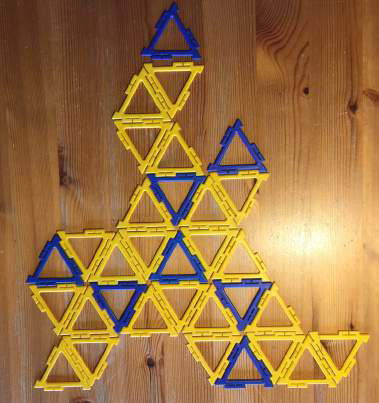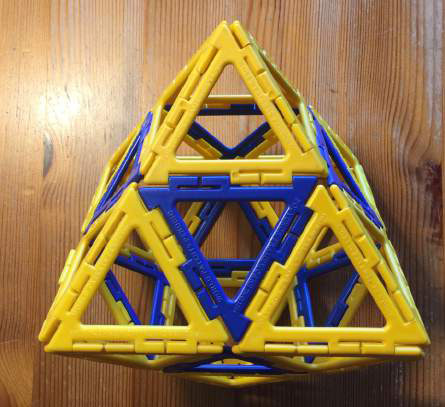The equatorial sections are now hexagons. There is one for each pair of opposite faces, 8/2 = 4 in all. We replace the yellow pyramids with squares and we have the solid 3.4.3.4: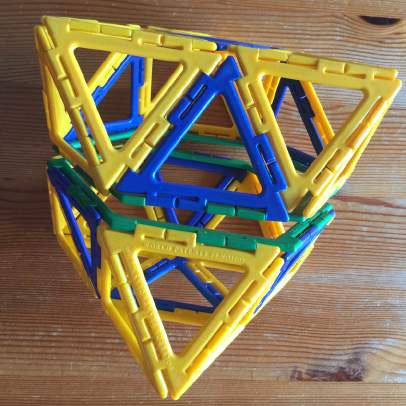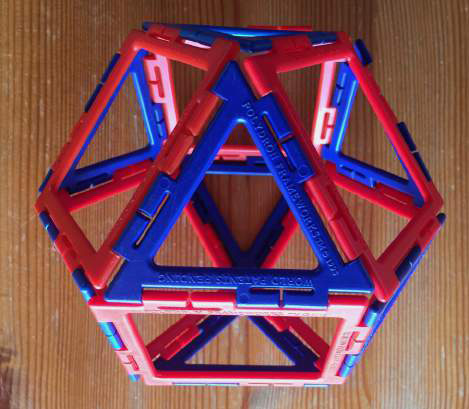There are 8 triangles and 6 squares. Calculating as before, we have v = 8 x 3/2 or 6 x 4/2 = 12, and an angle defect at each vertex of 720°/12 = 60°.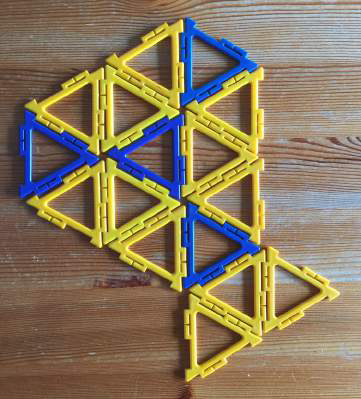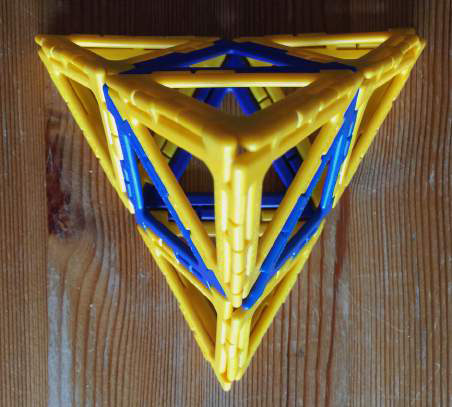## From 3.5.3.5 to 3.4.3.4

Back we go and remove yet a third yellow triangle. The angular defect is now 180° and the solid therefore has 720°/180° = 4 vertices. It is a tetrahedron: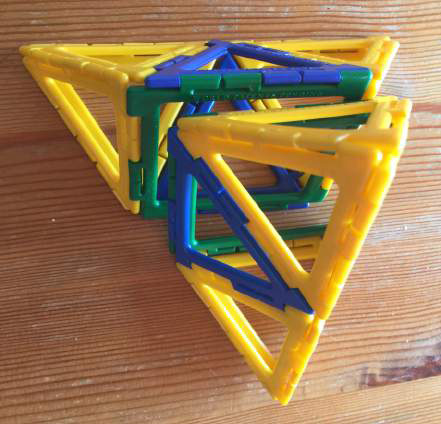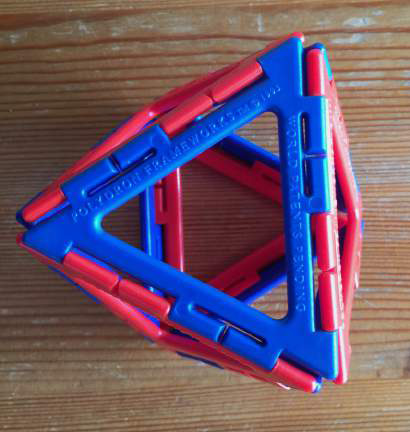The equatorial section is now a square. There is one for each pair of opposite edges, therefore 6/2 = 3 in all. The yellow pyramids are tetrahedra. Replacing each with a triangle, we have 3.3.3.3, the octahedron:

We know the angle defect for this solid, 120°.

## Conclusion

In this exercise we have taken a sequence of numerical strings, where the numbers represent the polygons which surround each vertex in a uniform tiling or solid, and seen what it means in terms of the geometry.

Paul Stephenson, Magic Mathworks Travelling Circus

 Share TweetTo contact us, call +44 (0) 1285 863980 or send us an email